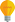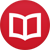# Are mathematical objects real?

by Jakub MarianTip: See my list of theMost Common Mistakes in English. It will teach you how to avoid mis­takes with com­mas, pre­pos­i­tions, ir­reg­u­lar verbs, and much more.

There are two major (and very different) “world views” among mathematicians. Adherents of Plato’s view believe that mathematical objects exist (as concepts) independently of our own intellect; that they are the only conceivable possibility of how any conceivable universe may work.

That, put just like that, would be a very bold statement, since existence of mathematical objects, as mathematicians understand the notion of existence, is based on the set of axioms we use. However, few mathematicians would dispute that. While real Platonists would try to seek one ultimate axiomatic theory that is the theory of the universe, most mathematicians understand that different axiomatic theories can be useful to model different physical processes, albeit just approximately, and consider the whole set of various theories to be “the only natural mathematics”.

On the other hand, there is a view that all mathematics is just a social construct; that it is no more or less natural than, for example, the concept of language. Human languages developed naturally as a means of communication and of thought. Why are they so well suited to everyday life? After hundreds of thousands of years of development, it would be a wonder if they weren’t. They developed in a sort of Darwinian manner; those constructions (and even whole languages) that were not useful to their speakers died out, while useful mutations persisted.

The same applies to mathematics. The first developed mathematical notion was that of natural numbers (that’s why they are called natural, isn’t it). Recognition of small natural numbers is actually hardwired into our brains; there are neural circuits able the recognize the number of objects in groups of up to about 4 objects (after that threshold, your subconscious just tells you that there are “many” objects); if you see a group of 3 objects, you know that there are exactly three objects without having to use any conscious process. That’s not surprising, because being able to tell two predators from three predators can be quite useful in a fight-or-flight decision-making process.

As human society developed, it became useful to be able to count. For practical purposes, it was obvious that if you have a certain amount of, say, apples, and someone gives you another apple, then you have even more apples, which is certainly good for you, and so the concept of counting was born. But what if someone wanted to give you two baskets of apples in exchange for one bigger basket of yours? Should you agree with that? An individual (and a society) who could answer such questions correctly was more likely to survive than those who could not, and so people learned to use elementary arithmetics.

## The grammar of mathematics

This process is not dissimilar to development of languages. There was most certainly nothing like declensions and tenses during the very first stage of development of human language; they developed over time because they were useful.

You may raise an objection that the origin of mathematics and that of language may be similar but that mathematics is based on very simple axioms, and everything else is derived from them, unlike in natural languages. First, let us note that although modern mathematics is based on simple axioms, actual mathematical theories are based on our intuition of usefulness. We can postulate any axioms we wish, but we choose only some specific sets of axiomsthose that yield a theory we find useful.

Secondly, axioms provide just the grammar we use, and we have quite a lot of freedom even within a certain set of axioms. I can define, for example, a function M by M(n) = “the number of ‘holes’ in the decimal representation of n”. For example M(1) = 0, M(6) = 1, M(8) = 2, M(69) = 2. Now, I can start developing a theory around it; for example if & denotes the operation of “concatenation” of numbers (e.g. 59 & 48 = 5948), then M(m & n) = M(m) + M(n). We have a sort of homomorphism of additive structures, so we must definitely be getting somewhere!

The fact is that anyone with at least rudimentary common sense will see that this notion is completely useless. As in English, even though the sentence “refrigerated unicorns will have squeezed the jelly out by Christmas” is grammatically correct, it is completely useless (and I am pretty sure I am the first person in the world who has used exactly this combination of words).

On the other hand, if I were the first to define φ(n) = “the number of natural numbers less than n which are relatively prime to n” and started developing a theory of it by saying that φ(n ⋅ m) = φ(n) ⋅ φ(m) for n and m relatively prime, I would be pretty famous by now, although from a layman’s view the two definitions could look similarly gibberish. Why would I be famous? Because φ has properties mathematicians find useful.

Similarly, why do people keep saying the sentence “a picture is worth a thousand words”? Because they find the information it provides useful. The word useful is the key here. We shape the whole mathematical theory, including the axioms it is based on, according to what we find useful.

The problem is that there is no objective sense of usefulness. We find certain things useful only because we are evolutionarily predisposed to find them useful.

## Existence of mathematical objects

Returning to the original question of existence of mathematical objectsI do not believe that mathematical objects as we define them should in any way be considered absolute or existent in any greater sense than as constructs of our own, and I would go as far as saying that even natural numbers and the very notion of logic and axiomatics are only artificial constructs of our evolutionarily predisposed minds.

I also believe that some of the deepest problems physicists face today are in fact only a product of our insufficient notion of mathematics. Think of the following: according to quantum mechanics, there is nothing like discrete objects in the world. Each particle is with certain probability just about everywhere, and we cannot tell where until we measure its position (which in fact also gives us just a probability distribution, only narrower).

So we see a sort of “non-discreteness” or “continuity” that leads to random results (with a certain distribution) when we try to “measure it”, which in fact means “comprehend it using our discrete notions” (numbers and discrete symbols). As a matter of fact, nobody has even yet been able to provide a satisfactory explanation of what a “measurement” in quantum mechanics is, and it may well be impossible to explain it using our discrete understanding of the universe.

It would be even naive to think so; why should a language that was originally created to perform tasks like counting apples be well suited to explanation of some of the deepest questions of our universe? Isn’t it a bit like trying to describe Mona Lisa using a thousand words? The point isif our mathematics is not objective but rather a construct of one particular species, it doesn’t make much sense to say that mathematical objects as we define them exist in any objective sense.

Let’s discuss that in greater detail in my article Do natural numbers exist?.

By the way, I have written several educational ebooks. If you get a copy, you can learn new things and support this website at the same time—why don’t you check them out?